# VolumetricPage 1

#### WATCH ALL SLIDES

Slide 1Volumetric ANALYSIS/TITRATION

Slide 2## Introduction

Titration is a common laboratory method of quantitative chemical analysis that is used to determine the unknown concentration of a known reactant. Because volume measurements play a key role in titration, it is also known as volumetric analysis.

Slide 3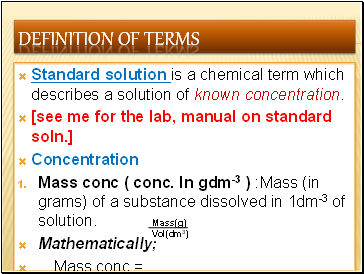## Definition of terms

Standard solution is a chemical term which describes a solution of known concentration.

[see me for the lab, manual on standard soln.]

Concentration

Mass conc ( conc. In gdm-3 ) :Mass (in grams) of a substance dissolved in 1dm-3 of solution.

Mathematically;

Mass conc =

Mass(g)

Vol(dm3)

Slide 4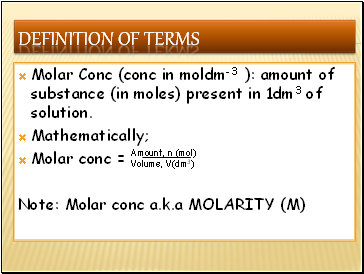Definition of terms

Molar Conc (conc in moldm-3 ): amount of substance (in moles) present in 1dm3 of solution.

Mathematically;

Molar conc =

Note: Molar conc a.k.a MOLARITY (M)

Amount, n (mol)

Volume, V(dm3)

Slide 5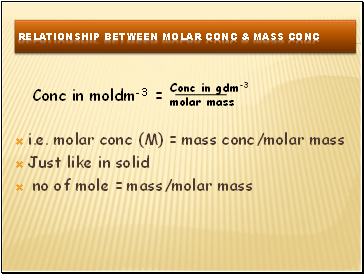## Relationship between molar conc & mass conc

i.e. molar conc (M) = mass conc/molar mass

Just like in solid

no of mole = mass/molar mass

Conc in moldm-3 =

Conc in gdm-3 molar mass

Slide 6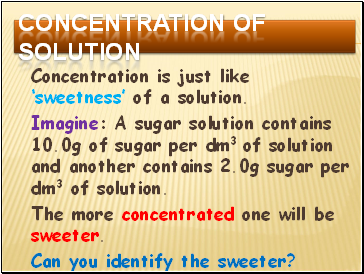## Concentration of solution

Concentration is just like ‘sweetness’ of a solution.

Imagine: A sugar solution contains 10.0g of sugar per dm3 of solution and another contains 2.0g sugar per dm3 of solution.

The more concentrated one will be sweeter.

Can you identify the sweeter?

Slide 7now it follows that:

The conc. of a solution is directly proportional to the amount(mole,n) of substance in solution at constant volume. C α n (V constant).

The conc. (c) of a soln. is inversely proportional to the vol(V) of soln, if the amount(mole/mass) is constant. C α 1/v (n constant).

V V V V V

C C C C C

Slide 8## Solved problems involved concentration

A solution contains 2.65g of anhydrous Na2CO3 in 200cm3 of solution. Calculate the conc. of the soln in gdm-3 [Na2CO3 = 106]

Hint: Do you notice that the problem is given in 2.65g per 200cm 3 ?.

Good!

Just express it in gdm-3 .

I mean gram in 1000cm3

SIMPLE!

Slide 9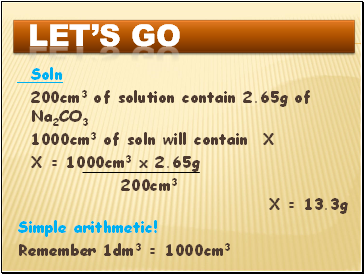let’s go

Go to page:
1  2  3  4  5  6  7